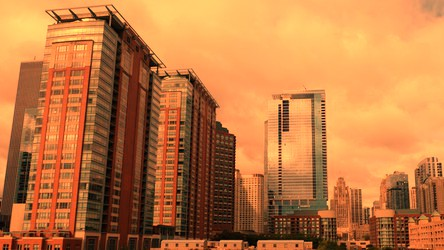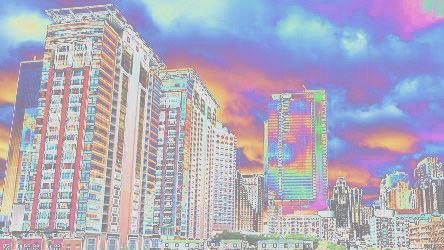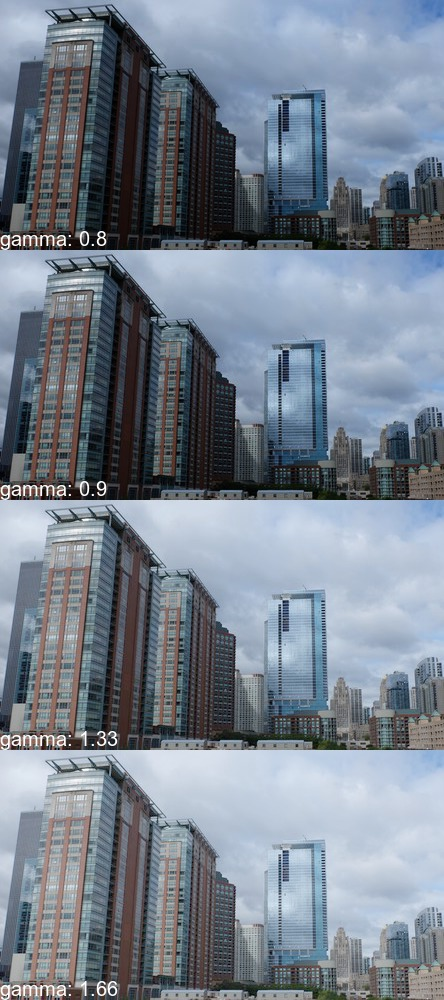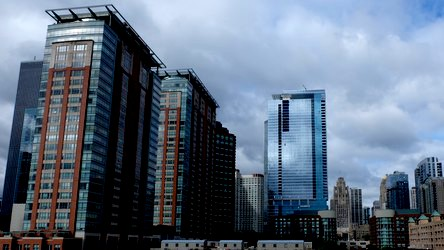# Color Enhancement¶

## Evaluate Expression¶

New in version 0.4.1.

Pixel channels can be manipulated by applying an arithmetic, relational, or logical expression. See EVALUATE_OPS for a list of valid operations.

For example, when given image enhancement.jpg:We can reduce the amount of data in the blue channel by applying the right-shift binary operator, and increase data in the right channel with left-shift operator:

from wand.image import Image

with Image(filename='enhancement.jpg') as img:
# B >> 1
img.evaluate(operator='rightshift', value=1, channel='blue')
# R << 1
img.evaluate(operator='leftshift', value=1, channel='red')## Function Expression¶

New in version 0.4.1.

Similar to evaluate(), function() applies a multi-argument function to pixel channels. See FUNCTION_TYPES for a list of available function formulas.

For example, when given image enhancement.jpg:We can apply a Sinusoid function with the following:

from wand.image import Image

with Image(filename='enhancement.jpg') as img:
frequency = 3
phase_shift = -90
amplitude = 0.2
bias = 0.7
img.function('sinusoid', [frequency, phase_shift, amplitude, bias])## Gamma¶

New in version 0.4.1.

Gamma correction allows you to adjust the luminance of an image. Resulting pixels are defined as pixel^(1/gamma). The value of gamma is typically between 0.8 & 2.3 range, and value of 1.0 will not affect the resulting image.

The level() method can also adjust gamma value.

For example, when given image enhancement.jpg:We can step through 4 pre-configured gamma correction values with the following:

from wand.image import Image

with Image(filename='enhancement.jpg') as img_src:
for Y in [0.8, 0.9, 1.33, 1.66]:
with Image(img_src) as img_cpy:
img_cpy.gamma(Y)## Level¶

New in version 0.4.1.

Black & white boundaries of an image can be controlled with level() method. Similar to the gamma() method, mid-point levels can be adjusted with the gamma keyword argument.

The black and white point arguments are expecting values between 0.0 & 1.0 which represent percentages.

For example, when given image enhancement.jpg:We can adjust the level range between 20% & 90% with slight mid-range increase:

from wand.image import Image

with Image(filename='enhancement.jpg') as img:
img.level(0.2, 0.9, gamma=1.1)
img.save(filename='enhancement-level.jpg')